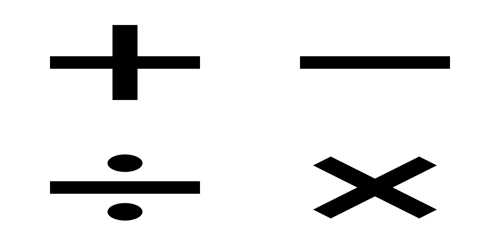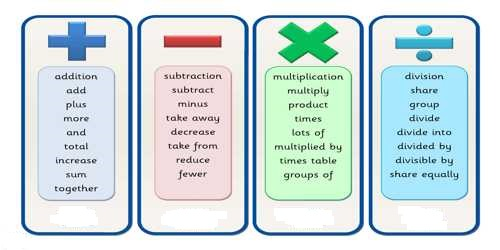Mathematic

# Basic Operations Terms Relating to MathematicsBasic Operations Terms Relating to Mathematics

Operations are actions or procedures which produce a resulting value from one or more input values.

The four basic mathematical operations are: Addition, subtraction, multiplication and division. The basic arithmetic properties are the commutative, associative, and distributive properties.

Addition is an operation indicated with + sign. The result of addition of two or more numbers (addends) is called their Sum

Subtraction is an operation indicated with a – sign. The result of a subtraction is called the difference.

Multiplication is an operation with * or dot or X or variables can simply be written together. The cross (X) is not normally used with literal numbers because it can be confused with the variable x. 5xy means 5 times x times y and is read 5 x, y. The result of a multiplication is called the product and the numbers that are multiplied together are the factors.

Division is an operation indicated by viniculum, slash or an obelus (÷) which is also know as a division sign. To divide x by y, where y is not zero, means to find a number z such that x = yz. x is called the dividend, y is called the divisor and z is called the quotient.Here define some other important Operations Terms:

Binary operations involve two quantities and include addition, subtraction, multiplication, division and exponentiation.

Unary operations only involve one quantity such as negation, absolute value or trigonometric functions.

Fundamental operations of Algebra are addition, subtraction, multiplication and division.

A Power is an operation indicated by a superscipt.

A Root is an operation indicated by the radical sign with a viniculum over the radicand.

Information Source: